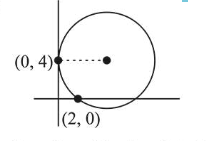# A circle touches the y-axis at the pointQuestion:

A circle touches the $y$-axis at the point $(0,4)$ and passes through the point $(2,0)$. Which of the following lines is not a tangent to this circle?

1. (1) $4 x-3 y+17=0$

2. (2) $3 x-4 y-24=0$

3. (3) $3 x+4 y-6=0$

4. (4) $4 x+3 y-8=0$

Correct Option: , 4

Solution:Equation of family of circle

$(x-0)^{2}+(y-4)^{2}+\lambda x=0$

Passes through the point $(2,0)$ then

$4+16+2 \lambda=0 \Rightarrow \lambda=-10$

Hence, the equation of circle

$x^{2}+y^{2}-10 x-8 y+16=0$

$\Rightarrow(x-5)^{2}+(y-4)^{2}=25$

Centre $(5,4)$.

$R=\sqrt{\frac{1}{2} \text { coeff. of } x+\frac{1}{2} \text { coeff. of } y \text {-constant }}$

$=\sqrt{25+16-16}=5$

Perpendicular distance of $4 x+3 y-8=0$ from the centre of circle

$=\left|\frac{20+16-8}{\sqrt{16+9}}\right|=\frac{28}{5} \neq 5$

Hence, $4 x+3 y-8=0$ can not be tangent to the circle.